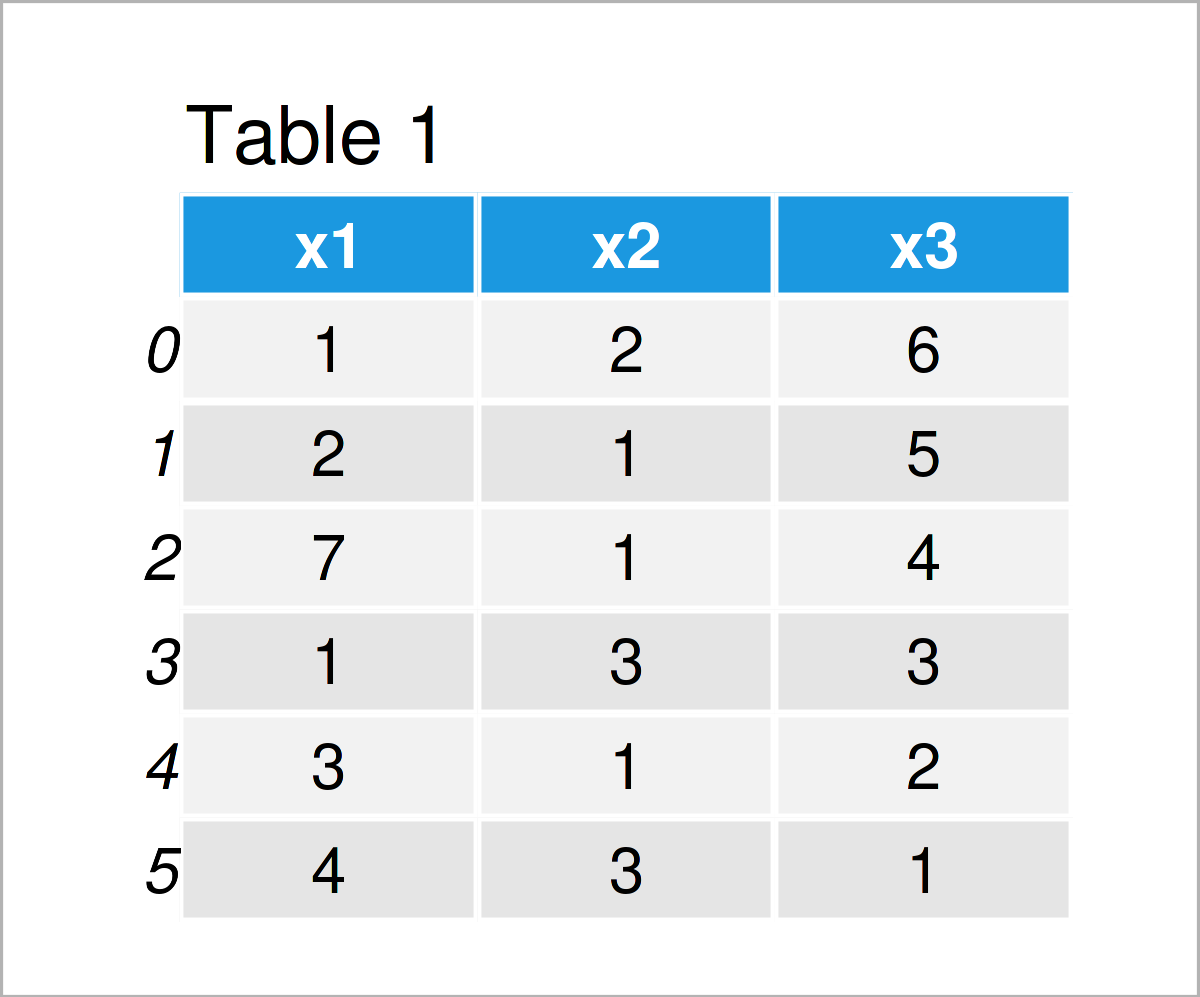# Determine if Value Exists in pandas DataFrame in Python (Example)

This tutorial explains how to check whether a specific value is contained in a pandas DataFrame in the Python programming language.

So now the part you have been waiting for – the Python code:

## Example Data & Add-On Libraries

First, we need to load the pandas library:

`import pandas as pd # Load pandas library`

We’ll use the following data as basement for this Python tutorial:

```data = pd.DataFrame({'x1':[1, 2, 7, 1, 3, 4], # Create example DataFrame 'x2':[2, 1, 1, 3, 1, 3], 'x3':range(6, 0, - 1)}) print(data) # Print example DataFrame```Table 1 shows the structure of our example data: It consists of six rows and three columns called “x1”, “x2”, and “x3”.

## Example: Check if Value Exists in pandas DataFrame Using values Attribute

The following Python programming syntax shows how to test whether a pandas DataFrame contains a particular number.

The following Python code searches for the value 5 in our data set:

```print(5 in data.values) # True```

As you can see based on the previous console output, the value 5 exists in our data.

Let’s check for the value 10:

```print(10 in data.values) # False```

The Python console returns the logical indicator False, i.e. the value 10 is not contained in our data matrix.

## Video, Further Resources & Summary

As an additional resource, I recommend watching the following video on the YouTube channel of Noureddin Sadawi. He explains how to select rows of a pandas DataFrame that have a certain value:

Please accept YouTube cookies to play this video. By accepting you will be accessing content from YouTube, a service provided by an external third party.If you accept this notice, your choice will be saved and the page will refresh.

At this point you should know how to test and determine whether a specific value is contained in a pandas DataFrame in Python. In case you have additional questions, let me know in the comments section. Furthermore, please subscribe to my email newsletter to get updates on the newest articles.

Subscribe to the Statistics Globe Newsletter